Home PHYSICS TOPIC 3: LIGHT | PHYSICS FORM 3

# TOPIC 3: LIGHT | PHYSICS FORM 3

2939
212## ​​​LIGHT PART I​​

​​ Reflection of Light from Curved Mirrors ​​ Types of curved mirrors  ​​​​

<> Convex  ​​ ​​ ​​ ​​ ​​ ​​​​ (diverging mirror) ​​

<> Concave  ​​ ​​ ​​​​ (converging mirror) ​​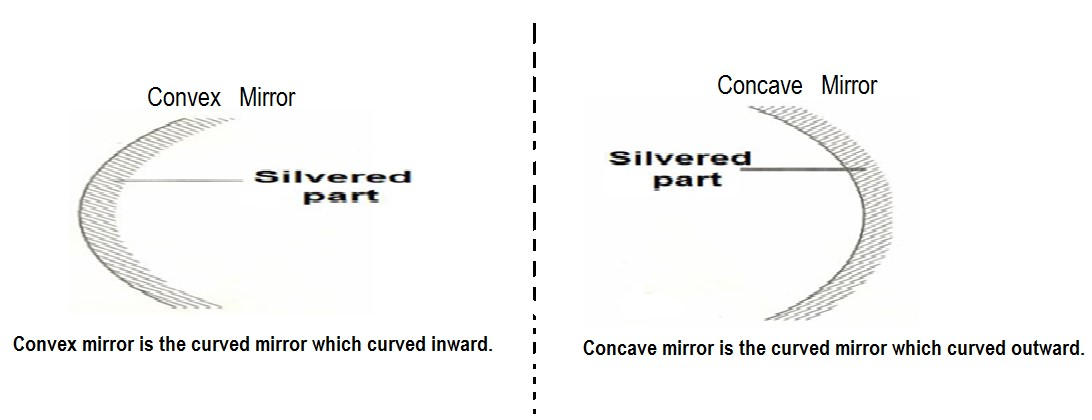​​ Terms used in curved mirrors​​

Consider the diagram below when two curved mirrors are joined ​​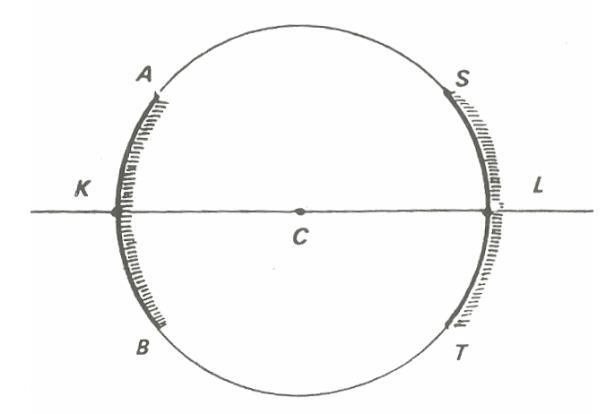​​ Whereby:​​ ​​

AB = Convex mirror  ​​​​ while ​​ ​​​​ ST = Concave mirror ​​

C = centre of curvature ​​

L = pole of the Concave mirror  ​​​​ while ​​ ​​​​ K = pole of the Convex mirror ​​

CL and CK are radii of curvature of Concave mirror and convex mirror respectively​​

CL and CK are principal axes of Concave and Convex mirror respectively​​

Centre of Curvature:  ​​ ​​ ​​​​ Is the centre of the sphere in which the mirror is a part. ​​ Radius of Curvature:  ​​ ​​ ​​ ​​​​ Is the distance or length between the pole of the curved mirror and the centre of curvature. ​​

Principal Axis: ​​ Is the line joining the pole of the curved mirror and the centre of curvature. ​​

Consider when light is reflected in curved mirrors as shown in the diagrams below. ​​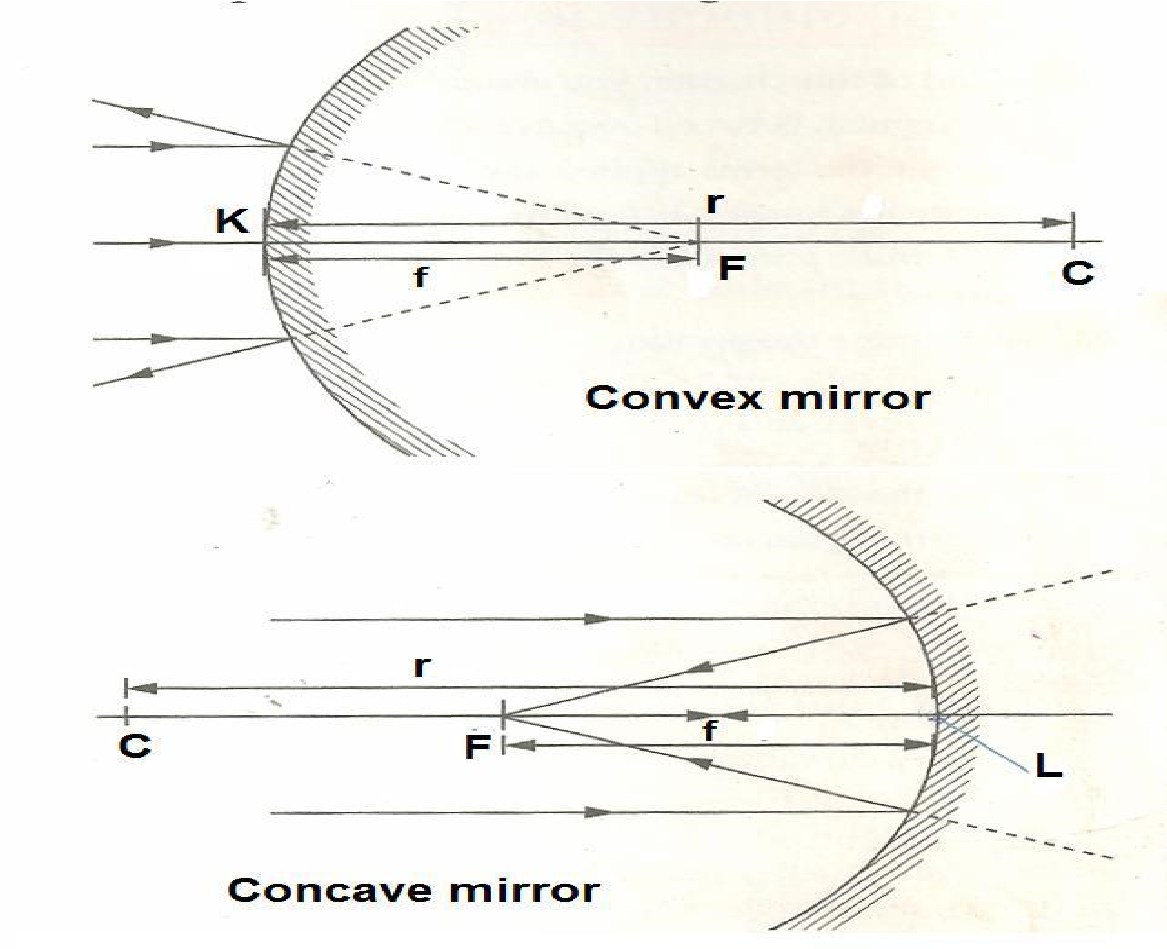​​ Principle Focus, F: ​​ Is the point in which the light is reflected in curved mirror ​​

Focal Length, f  ​​ ​​​​ Is the distance between pole of the curved mirror and the principal focus.  ​​ ​​ ​​ ​​ ​​ ​​ ​​ ​​ ​​ ​​ ​​ ​​ ​​ ​​ ​​ ​​ ​​ ​​ ​​​​ NB:  ​​ ​​ ​​ ​​ ​​ ​​ ​​ ​​ ​​ ​​ ​​ ​​ ​​ ​​ ​​ ​​ ​​ ​​ ​​ ​​ ​​ ​​​​ Given that focal length is always half the radius of curvature.​​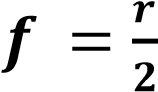​​ Location of Image using Ray diagrams​​ ​​

The following are the rules used to locate image in the curved mirror. ​​

A ray of light travelling to the mirror parallel to the principal axis, a ray is reflected through the principal focus ​​

A ray of light travelling to the mirror through the centre of curvature is reflected along its own path ​​

A ray of light travelling to the mirror through the principal focus is reflected parallel to the principal axis ​​

Note:​​ Any two of these rays are sufficient to locate the image. ​​

​​ Procedure to draw ray diagrams​​ ​​ o​​ Choose an appropriate scale so that the ray diagram fits on the available space. ​​ o​​ Draw a horizontal line to represent the principal axis of the mirror. Mark the focal point of the mirror. ​​

Using the chosen scale, draw the object in position along the principal axis. The object is drawn as a vertical line from the principal axis. ​​

Locate the position of the image by drawing rays from the object to the mirror. Use the rules for drawing ray diagrams to draw the reflected rays. ​​

At the point of intersection of the reflected rays, draw the image in position ​​

Image formed in Curved mirror ​​

Terms used to describe the images formed by curved mirrors: ​​

Position​​

Real image​​ is on the same side of the mirror as the object. ​​

Virtual image​​ is on the opposite side of the mirror compared to the object. ​​

Nature​​ ​​

Upright image​​ has the same orientation as the object. ​​

Inverted image​​ is oriented in an upside down position compared to the object. ​​

Size​​ ​​

Enlarged image​​ is bigger than the object. ​​

Diminished image​​ is smaller than the object ​​

Images formed by Concave mirrors​​ ​​

The following are the characteristics of images formed by concave mirrors: ​​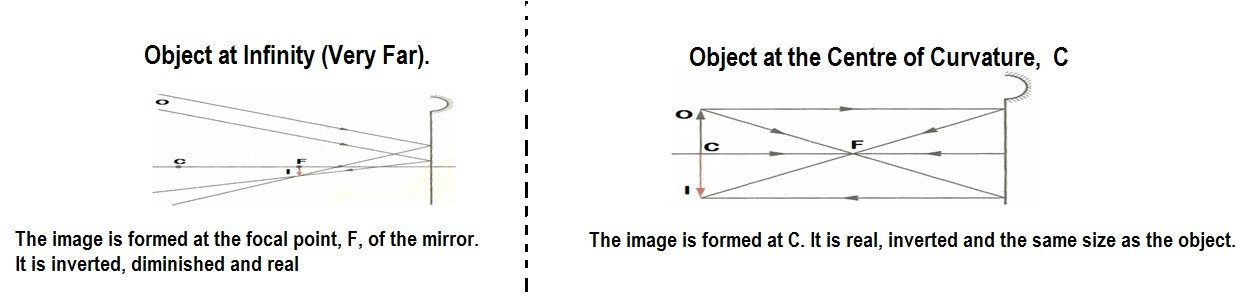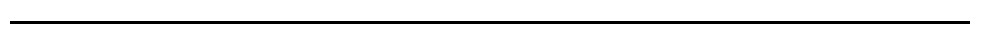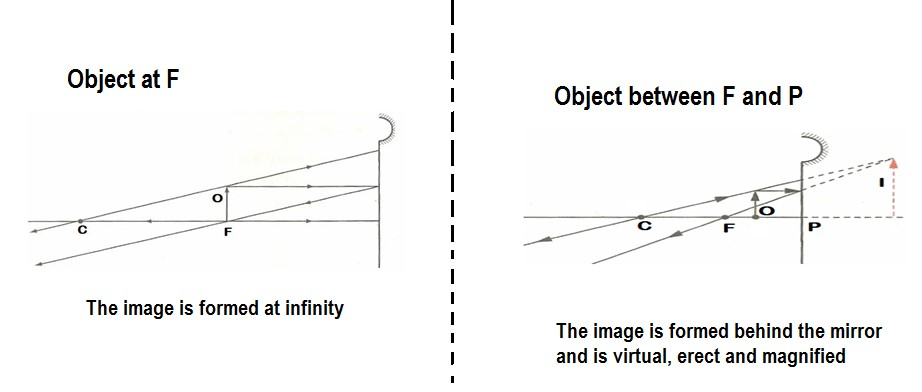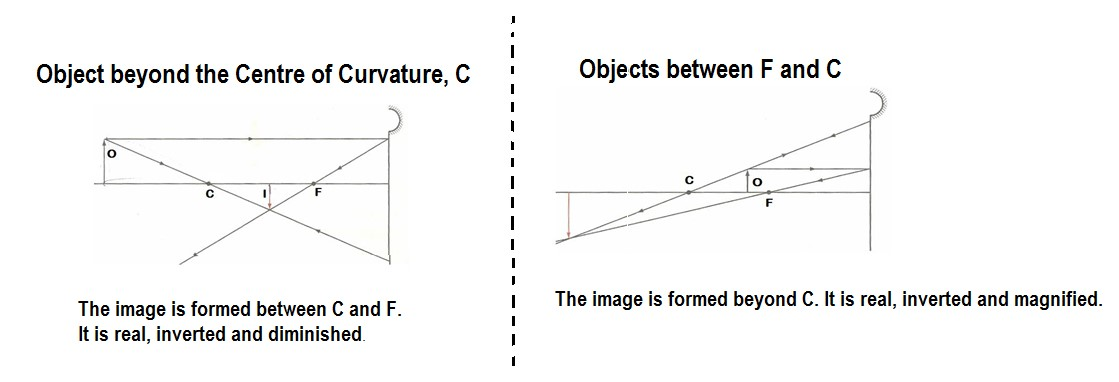2. Example​​

​​ An object 5 cm tall is placed 34 cm from a concave mirror of focal length 20 cm. By means of an accurate graphical construction, determine the position, size and the  ​​ ​​ ​​ ​​ ​​ ​​ ​​ ​​ ​​​​ nature of the image formed.​​